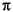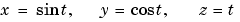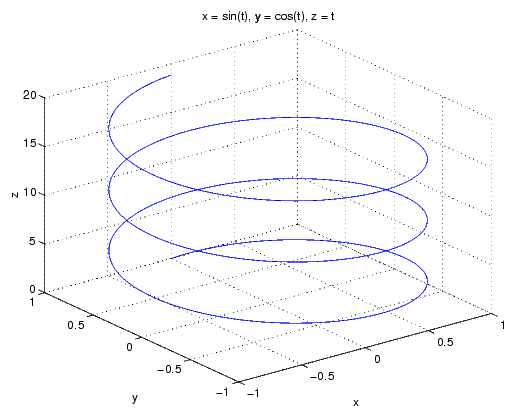MATLAB Function Referenceezplot3

Easy to use 3-D parametric curve plotter

Syntax

• ```ezplot3(funx,funy,funz)
ezplot3(funx,funy,funz,[tmin,tmax])
ezplot3(...,'animate')
ezplot3(axes_handle,...)
h = ezplot3(...)
```

Description

`ezplot3(funx,funy,funz)` plots the spatial curve `funx(t)`, `funy(t)`, and `funz(t)` over the default domain 0 < `t` < 2.

`funx`, `funy`, `and` `funz` can be function handles for M-file functions or an anonymous functions (see Function Handles and Anonymous Functions) or strings (see the Remarks section).

```ezplot3(funx,funy,funz,[tmin,tmax]) ``` plots the curve `funx(t)`, `funy(t)`, and `funz(t)` over the domain `tmin` < `t` < `tmax`.

```ezplot3(...,'animate') ``` produces an animated trace of the spatial curve.

```ezplot3(axes_handle,...) ``` plots into the axes with handle `axes_handle` instead of the current axes (`gca`).

```h = ezplot3(...) ``` returns the handle to the plotted objects in `h`.

Remarks

Passing the Function as a String

Array multiplication, division, and exponentiation are always implied in the expression you pass to `ezplot3`. For example, the MATLAB syntax for a plot of the expression

• ```x = s./2, y = 2.*s, z = s.^2;
```

which represents a parametric function, is written as

• ```ezplot3('s/2','2*s','s^2')
```

That is, `s/2` is interpreted as `s./2` in the string you pass to `ezplot3`.

Passing a Function Handle

Function handle arguments must point to functions that use MATLAB syntax. For example, the following statements define an anonymous function and pass the function handle `fh` to `ezplot3`.

• ```fh1 = @(s) s./2; fh2 = @(s) 2.*s; fh3 = @(s) s.^2;
ezplot3(fh1,fh2,fh3)
```

Note that when using function handles, you must use the array power, array multiplication, and array division operators (`.^, .*, ./`) since `ezplot` does not alter the syntax, as in the case with string inputs.

Passing Additional Arguments

If your function has additional parameters, for example `k` in `myfuntk`:

• ```function s = myfuntk(t,k)
s = t.^k.*sin(t);
```

then you can use an anonymous function to specify that parameter:

• ```ezplot3(@cos,@(t)myfuntk(t,1),@sqrt)
```

Examples

This example plots the parametric curve

•over the domain [0,6]:

• ```ezplot3('sin(t)','cos(t)','t',[0,6*pi])```

See Also

`ezplot`, `ezpolar`, `function_handle`, `plot3`

Function Plots for related functions

© 1994-2005 The MathWorks, Inc.